﻿ 基于灰狼优化器改进蚁群算法的物流配送路径优化算法

基于灰狼优化器改进蚁群算法的物流配送路径优化算法Logistics Distribution Route Optimization Algorithm Based on Improved Ant Colony Algorithm of Gray Wolf Optimizer

Abstract: In solving multi task logistics distribution route optimization, ant colony algorithm has some problems, such as lack of standard parameter setting and different parameter setting in different situations. This paper proposes an improved ant colony algorithm based on gray wolf optimizer. In view of the uncertain parameter setting of traditional ant colony algorithm, the gray wolf optimizer is introduced to find the optimal parameters of ant colony by virtue of the global search and progressive search characteristics of gray wolf optimizer, so as to automatically obtain the optimal parameters and solve the parameter configuration problem of ant colony algorithm. Finally, the improved algorithm is applied to the multi task logistics distribution path optimization. Experimental results show that the proposed algorithm can automatically get better parameters, and the algorithm has good accuracy and robustness.

1. 引言

Mirjalili等人  受灰狼群体捕猎行为的启发，于2014年提出一种灰狼优化算法(Grey Wolf Optimization, GWO)算法，该算法作为一种新兴的群智能元启发式算法，通过借鉴灰狼的社会组织与狩猎时的行为模式，使得算法具有很强的搜索能力。目前，该算法在众多工程领域获得了广发应用，包括车间调度、数值优化、图像融合以及电力系统配置等。文献  中，借助A*算法来初始化头狼，让灰狼优化器能有一个更好的起点。文献  在GWO的基础上提出了HGWO算法，借助反向学习的方法，提升初始种群的质量，增强算法的勘探能力。文献  中提出了使用HGWO自动适应VMD的方法，提高了原有模型的精度。文献  通过引入了正余双弦函数，来控制其收敛速度，提高了原有模型的收敛精度，一定程度上避开了过拟合。文献  中，通过引入非线性惯性权重和交叉变异策略，强化全局搜索能力和多约束条件搜索能力。文献  是基于传统的算法提出的AGWO算法，该算法引入了弹性机制、循环机制和攻击机制，改进后的算法在局部最优回避和计算精度上明显由于其它启发式算法。在软件方面，文献  提出了基于GWO的ARP-GWO算法，对风险进行优先级排序，使用集成指标和可用性指标，让软件开发可以节约更多成本，短时间内交付出更高质量的产品。

2. 环境建模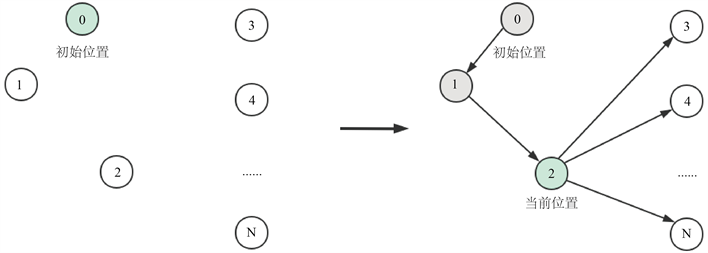Figure 1. User simulation mobile model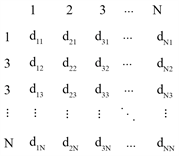Figure 2. Distance relationship among N cities

3. 相关算法

3.1. 蚁群算法

${p}_{ij}^{k}=\left\{\begin{array}{l}\frac{{\tau }_{ij}^{\alpha }\left(t\right)\ast {\eta }_{ij}^{\beta }\left(t\right)}{{\sum }_{s\in allowe{d}_{k}}{\tau }_{ij}^{\alpha }\left(t\right)\ast {\eta }_{ij}^{\beta }\left(t\right)},\text{\hspace{0.17em}}\text{\hspace{0.17em}}j\in allowe{d}_{k}\\ 0,\text{\hspace{0.17em}}\text{\hspace{0.17em}}\text{\hspace{0.17em}}\text{\hspace{0.17em}}\text{\hspace{0.17em}}\text{\hspace{0.17em}}\text{\hspace{0.17em}}\text{\hspace{0.17em}}\text{\hspace{0.17em}}\text{\hspace{0.17em}}\text{\hspace{0.17em}}\text{\hspace{0.17em}}\text{\hspace{0.17em}}\text{\hspace{0.17em}}\text{\hspace{0.17em}}\text{\hspace{0.17em}}\text{\hspace{0.17em}}\text{\hspace{0.17em}}\text{\hspace{0.17em}}\text{ }\text{ }\text{\hspace{0.17em}}\text{\hspace{0.17em}}\text{\hspace{0.17em}}\text{\hspace{0.17em}}\text{\hspace{0.17em}}\text{\hspace{0.17em}}\text{\hspace{0.17em}}\text{\hspace{0.17em}}\text{\hspace{0.17em}}\text{\hspace{0.17em}}\text{\hspace{0.17em}}\text{\hspace{0.17em}}\text{\hspace{0.17em}}j\notin allowe{d}_{k}\end{array}$ (1)

${\tau }_{ij}\left(t+1\right)={\tau }_{ij}\left(t\right)\ast \left(1-\rho \right)+\Delta {\tau }_{ij}$ (2)

$\Delta {\tau }_{ij}=\underset{k=1}{\overset{m}{\sum }}\Delta {\tau }_{ij}\left(k\right)$ (3)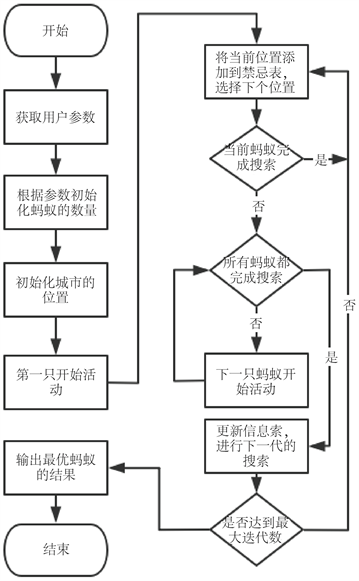Figure 3. Flow chart of ant colony algorithm

3.2. 灰狼算法

$\stackrel{\to }{D}=|\stackrel{\to }{C}\ast \stackrel{\to }{{X}_{p}}\left(t\right)-\stackrel{\to }{X}\left(t\right)|$ (3)

$\stackrel{\to }{X}\left(t+1\right)=\stackrel{\to }{{X}_{p}}\left(t\right)-\stackrel{\to }{A}\ast \stackrel{\to }{D}$ (4)

$\stackrel{\to }{A}=2\stackrel{\to }{a}\ast \stackrel{\to }{{r}_{1}}-\stackrel{\to }{a}$ (5)

$\stackrel{\to }{C}=2\ast \stackrel{\to }{{r}_{2}}$ (6)

$\stackrel{\to }{{D}_{\alpha }}=|\stackrel{\to }{{C}_{1}}*\stackrel{\to }{{X}_{\alpha }}-\stackrel{\to }{X}|,\stackrel{\to }{{D}_{\beta }}=|\stackrel{\to }{{C}_{1}}*\stackrel{\to }{{X}_{\beta }}-\stackrel{\to }{X}|,\stackrel{\to }{{D}_{\delta }}=|\stackrel{\to }{{C}_{1}}*\stackrel{\to }{{X}_{\delta }}-\stackrel{\to }{X}|$ (7)

$\stackrel{\to }{X}\left(t+1\right)=\frac{\stackrel{\to }{{X}_{1}}+\stackrel{\to }{{X}_{2}}+\stackrel{\to }{{X}_{3}}}{3}$ (8)

4. 基于灰狼优化器改进的蚁群算法

$a=2\mathrm{sin}\left(\frac{\pi }{2}\left(\text{1}-\frac{t}{\text{Max_iter}}\right)\right)$ (9)

$\text{fitness}=\left\{\begin{array}{l}\mathrm{min}\left(\text{consuming}\right);路径长相等\\ \mathrm{min}\left(\text{route}\right);\text{others}\end{array}$ (10)

$m\left(t+1\right)=\left\{\begin{array}{l}\text{Min}\left(m\left(t\right)\right),所有灰狼结果相等\\ \text{Mincons}\left(m\left(t\right)\right),出现更快收敛解\\ \text{fitness}\left(m\left(t\right)\right),出现新的最优解\end{array}$ (11)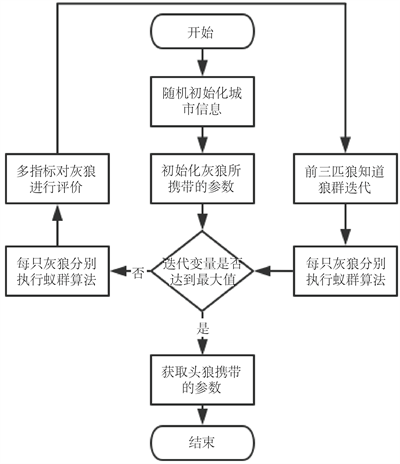Figure 4. Algorithm flow chart

Step1：随机初始化每个城市的位置，计算出每个城市之间的距离，并存入到邻接矩阵中，方便后续的计算。

Step2：初始化灰狼，让狼群均匀分布在解的空间内，设置预定的最大迭代数。

Step3：使用每只灰狼携带的参数分别运行蚁群算法，运行时要使用在Step(1)中预设的城市数据。

Step4：分析每只灰狼的运行结果，让结果最优，收敛最快的灰狼成为首领，指导狼群更新位置，继续搜索猎物。

Step5：当狼群达到预定的迭代数后停止继续迭代，获取头狼的坐标信息，从而得到最优的参数信息。

5. 实验结果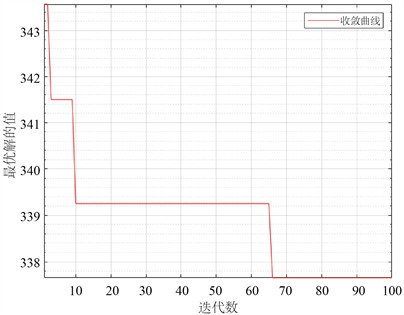Figure 5. The results of the improved algorithm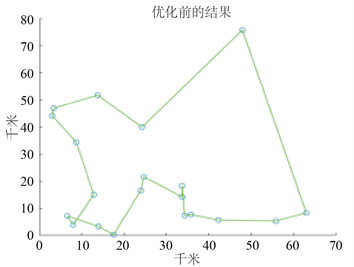(a) 优化前的效果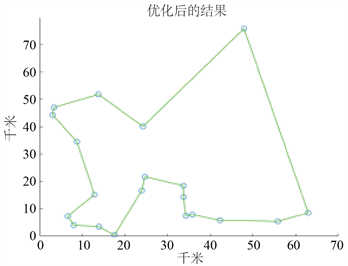(b) 优化后的效果

Figure 6. Operation results before and after optimizationFigure 7. Comparison of convergence curvesTable 1. Results of five experiments

6. 结语

NOTES

*通讯作者。

 李锋源, 许艳萍, 王武. 多策略蚁群算法求解机器人路径规划[J]. 福州大学学报(自然科学版), 2011, 39(3): 385-391.

 刘关俊. 基于粒子群算法的移动机器人路径规划研究[D]: [硕士学位论文]. 长沙: 中南大学, 2007.

 陈刚, 沈林成. 复杂环境下路径规划问题的遗传路径规划方法[J]. 机器人, 2001, 23(1): 40-44.

 张江霄. 基于混合扫描法的优化蚁群算法的物流配送系统研究与应用[Z]. 河北省, 邢台学院, 2019-07-18.

 刘玉霞, 王萍, 修春波. 基于模拟退火策略的逆向蚁群算法[J]. 微计算机信息, 2006, 22(34): 265-267.

 Li, S., You, X. and Liu, S. (2021) Multiple Ant Colony Optimization Using Both Novel LSTM Network and Adaptive Tan-imoto Communication Strategy. Applied Intelligence, No. 9, 1-21.
https://doi.org/10.1007/s10489-020-02099-z

 Guan, B., Zhao, Y. and Li, Y. (2021) An Improved Ant Colony Optimization with an Automatic Updating Mechanism for Constraint Satisfaction Problems. Expert Systems with Appli-cations, 164, Article ID: 114021.
https://doi.org/10.1016/j.eswa.2020.114021

 龚铭凡, 徐海祥, 冯辉, 薛学华. 基于改进蚁群算法的智能船舶路径规划[J]. 武汉理工大学学报(交通科学与工程版), 2020, 44(6): 130-134.

 肖艳秋, 焦建强, 乔东平, 等. 蚁群算法的基本原理及应用综述[J]. 轻工科技, 2018(3): 69-72.

 Mirjalili, S., Mirjalili, S.M. and Lewis, A. (2014) Grey Wolf Optimizer. Advances in Engineering Software, 69, 46-61.

 曹建秋, 张广言, 徐鹏. A*初始化的变异灰狼优化的无人机路径规划[J/OL]. 计算机工程与应用, 2021: 1-12. http://kns.cnki.net/kcms/detail/11.2127.TP.20201225.0932.014.html, 2021-04-08.

 王永琦, 江潇潇. 基于混合灰狼算法的机器人路径规划[J]. 计算机工程与科学, 2020, 42(7): 1294-1301.

 Gai, J., Shen, J., Hu, Y., et al. (2020) An Integrated Method Based on Hybrid Grey Wolf Optimizer Improved Variational Mode Decomposition and Deep Neural Network for Fault Diagnosis of Rolling Bearing. Measurement, 162, Article ID: 107901.
https://doi.org/10.1016/j.measurement.2020.107901

 石春花, 刘环. 基于正余双弦自适应灰狼优化算法的医药物流配送路径规划[J]. 数学的实践与认识, 2020, 50(14): 114-127.

 袁光辉. 基于改进灰狼优化算法的物流配送路径规划[J]. 荆楚理工学院学报, 2019(3): 12-19.

 Meng, X., Jiang, J. and Wang, H. (2021) AGWO: Advanced GWO in Multi-Layer Perception Optimization. Expert Systems with Applications, Article ID: 114676.
https://doi.org/10.1016/j.eswa.2021.114676

 Prakash, B. and Viswanathan, V. (2021) ARP-GWO: An Effi-cient Approach for Prioritization of Risks in Agile Software Development. Soft Computing, 25, 5587-5605.
https://doi.org/10.1007/s00500-020-05555-7

Top